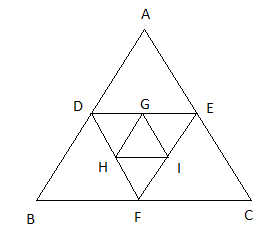# Elitmus previous papers set - 3

1. There is a equilateral triangle whose area is A.  Another triangle is formed by joining the mid points.  This process is continued.  Find the sum of these areas.
Explanation:Let ABC is an equilateral triangle with area = A
Remember:  The area of the triangle formed when mid points of the triangle is joined is exactly one-fourth of the bigger triangle. So Area of DEF = A/4.  Similarly Area of GHI = A/16 and so on ...
So Sum of the areas of all triangles = $A + \dfrac{A}{4} + \dfrac{A}{{16}} + ...$
$\Rightarrow A \left( {1 + \dfrac{1}{4} + \dfrac{1}{{16}} + ...} \right)$
The series in the bracket is G.P.  and ${S_\infty } = \dfrac{a}{{1 - r}}$
$\Rightarrow A\left( \vcenter{\dfrac{1}{{1 - \dfrac{1}{4}}}} \right)$ $\Rightarrow \dfrac{4}{3}A$

2. Probability of Cristiano Ronaldo scoring a penalty is twice than the probability of missing it. In a World cup he takes 5 penalties, What is the probability of scoring 3 penalties among the 5?
a) 5 *2 *((2/3)^3 * (1/3)^2)
b) 1-((2/3)^3 * (1/3)^2)
c) 1-((2/3)^2 * (1/3)^3)
d) None of the above.
Explanation:
We have to use Binomial distribution to solve this question as Ronaldo scoring penalty and not scoring penalty are Dichotomous in nature.
Success + Failure = 1
2x + x = 1
So x = 1/3
Probability of scoring a penalty = p = 2/3 and Not scoring it = q =  1/3
Probability of scoring exactly 'r' of 'n' trials = $P(x = r) = {}^n{C_r}.{p^r}.{q^{n - r}}$
$P(x = 3) = {}^5{C_3}.{\left( {\dfrac{2}{3}} \right)^3}.{\left( {\dfrac{1}{3}} \right)^{5 - 3}}$
= $10.{\left( {\dfrac{2}{3}} \right)^3}.{\left( {\dfrac{1}{3}} \right)^2}$

3. How many such 4 consecutive numbers are there less than 1000 when added gives a sum which is divisible by 10? For example, 16 +17+18+19=70.
Explanation:
Let the numbers be n, n+1, n+2, n+3.
Sum of these numbers = 4n + 6.
Let 4n + 6 = 10k
So n = $\dfrac{{10k - 6}}{4}$ = $2k - 1 + \dfrac{{2k - 2}}{4}$ = $2k - 1 + \dfrac{{k - 1}}{2}$
So k takes only odd numbers.
For k = 1, n = 1
For k = 3, n = 6
For k = 5, n = 11
. . . .
. . . .
Final value of n = 996.
So total values = $= \dfrac{{l - a}}{d} + 1 = \dfrac{{996 - 1}}{5} + 1$ = 200

4. 1,2,3,4,5,6,7 are arranged such that sum of two successive numbers is a prime number.  For example, 1234765 (i.e. 1+2=3, 2+3=5, 3+4=7....)
1.  How many such possible combinations occur?
2.  How many possible combination occurs if first number is 1/7 and last number is 7/1 (i.e 1xxxxx7 or 7xxxxx1)?
3.  How many numbers will come on 4th position(xxx_xxx)?
Explanation:
We have to follow a systematic approach for this question.  We know that if at all two consecutive numbers be prime, they should not be even in the first place.  So we arrange even numbers in even places, odd numbers in odd places.
2 should not have 7 as its neighbor
4 should not have 5 as its neighbor
6 should not have 3 as its neighbor
Case 1:
__ 2 ____ 4 ____    6 __
x7    x7,5      x5,3       x3
So fix, 3 or 1 in between 2 and 4. We get the following options with reverse case also.
5234167, 7614325
5234761, 1674325
1234765, 5674321
3214765, 5674123

Case 2:
__ 2 ____ 6 ____   4 __
x7    x7,3      x3,5      x5

5216743, 3476125
3256147, 7416523
3256741, 1476523
1256743, 3476521

Case 3:
__ 4 ____ 2 ____    6 __
x5    x5,7     x7,3       x3

3412567, 7652143
7432165, 5612347
7432561, 1652347
1432567, 7652341
Answer 1: Total possibilities are 24.